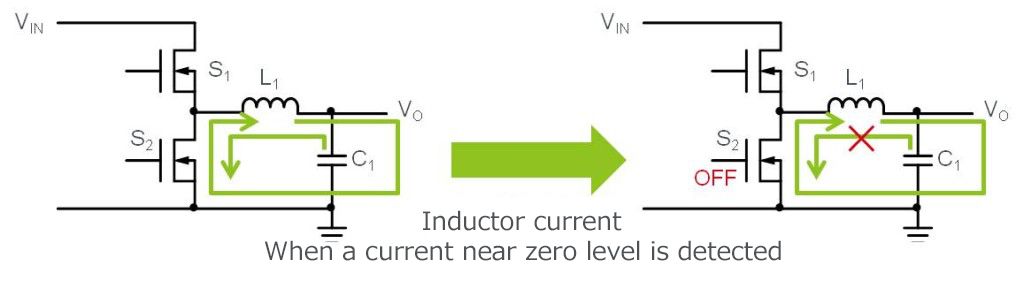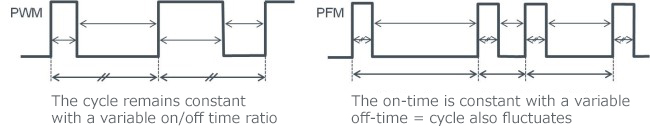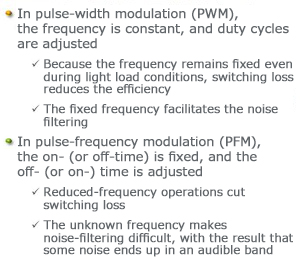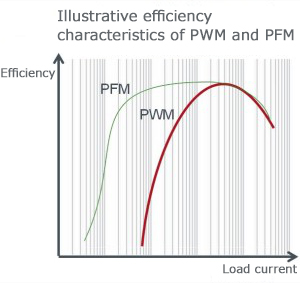# Efficiency Improvements at Light Load for the Synchronous Rectifying Type

2015.12.06

・In light load operations, in terms of standby efficiency the self-consumption of power becomes predominant; therefore, the operation must be shifted in the direction of reducing the self-power consumption.

・Because these functions are provided in the IC itself, basically no additional circuits or parts are needed.

・With respect to noise, in many cases it must be suppressed even at the expense of efficiency.

The preceding section explained that the synchronous type suffers from a decline in efficiency due to the reverse current flow during light load conditions. Given the fact that the synchronous type yields high efficiency, obviously it would be desirable if it could maintain high efficiency even during the light load conditions. Moreover, newer devices are expected to reduce standby power consumption significantly. The lightest load condition occurs when the circuit to which power is supplied is shut down. The problem would be solved if the power supply unit can also be shut down. However, minute power is needed on a continuous basis, and the low efficiency in that mode of operation presents a significant issue.

## Addition of a discontinuous mode

One of the strategies for improving the efficiency of the synchronous rectifying type involves the addition of a function that operates in discontinuous mode during the light load state. This is based on a simple concept in that when the condition of the inductor current falling to almost zero is detected, the lower-side transistor is turned off to prevent any reverse current flow from occurring (Figure 43).Figure 43

This strategy, however, is not without problems. Because in this operation the node on the transistor for the inductor assumes a condition equivalent to being open, any electric discharge from the output capacitor depends on the load current, and due to the fact that the load is light, it takes a long time for the voltage to decline. This reduces the switching speed and increases the ripple voltage in some cases.

To make the matter worse, the fact that the high side transistor does not turn on until the output voltage declines alters the switching cycle. For the filtering of noise, fluctuations of the noise frequency present a problem, which is also tradeoff with efficiency.

## Switching from PWM mode to PFM mode

Whereas the discussion up to this point has been predicated on the use of PWM, there is another approach involving the use of PWM and PFM to exploit the advantages inherent in each method. When the load is heavy, control is switched to PWM, and when the load is light, more efficient PFM takes over. PWM is a commonly employed voltage control method in which the frequency is held constant, such that whether the load is heavy or light and even if the on/off time ratio varies, the number of switching operations remains the same. Consequently, because the self-consumption of power does not change, at times of light load the switching loss becomes predominant, which reduces efficiency. This is the reason that in the PWM mode generally the efficiency decreases rapidly with light load.Figure 44

In PFM, the on-time is constant while the off-time varies. Or, the off-time remains constant and the off-time is variable (Figure 44 shows an example in which the on-time is fixed). In other words, the length of time until the switch is turned on next time varies. During light load conditions, the amount of additional power that must be supplied can be small, and consequently even when the cycle time is long the number of switching operations per unit time decreases, with the result that switching loss diminishes and favorable efficiency is maintained (see Figure 46).Figure 45Figure 46

The question, then, might be asked: “Why don’t we simply adopt the PFM method?” However, a variable on-cycle, that is, frequency, would make the noise stemming from switching operations indefinite. The resulting inability to identify frequency can make the noise-filtering process extremely difficult. Also, if noise enters the 20 kHz band, which is an audible band, the problem of ringing can occur, which produces an adverse impact on S/N in audio devices. As far as noise is concerned, PWM may be preferable in many respects. Consequently, this too is a matter of tradeoffs.